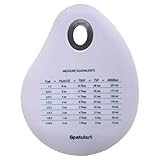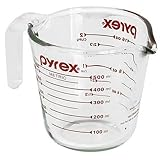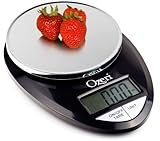Home

Compare Prices

Weight ConversionsQuick Lists

Complete List

Grocery Stores

New Store RequestsSuggest an Item

Suggest a Store

Future Plans

# Weight Conversions

## Introduction | US System | US vs. Metric SystemMost food products offer US and metric weight and volume information these days. We'll help you figure out how much you�re really buying.

### Compare Prices  >>>  GO!  >>>

For shoppers like us, weight conversion really means weight and volume conversion, because weight only applies to solid products such as bread, pizza, chocolate and so on.

Liquids, on the other hand, are not measured by weight but by volume instead. So when we talk about a gallon of milk, this measurement really refers the space occupied by the milk, not its weight.

Believe it or not, but a gallon of milk has a different weight than a gallon of water. This has to do with the fact that both liquids have different consistencies. (Think of a hand full of feathers and a hand full of lead. Both are a hand full, but I�m sure you�ll agree the lead is heavier.)

Below you will find two types of conversions: relational conversions and inter-system conversions.

The relational conversion shows how weight and volume units are related within the US system. After all, what exactly are 20 oz?

The inter-system conversion demonstrates how US measurements translate into metric units. Many items are imported from outside the US where the metric system is standard. This standard of measurement has been appearing more and more on our food packaging (just think of the 2 Liter coke bottle), so these conversions will help you get a handle on a product’s weight or volume when comparing it to other items.

Given our quest for usability of this site, we decided to stick only to the essential weights and volumes you�ll find on grocery packaging. Admittedly, some units you�ll find below could have been broken down even more, but this section has already spun out of control. Originally I expected not to jot down more than two to four lines�

### Abbreviations

lb :: pound

oz :: ounce

#### US Liquids (volume)

gal :: gallon

qt :: quart

pt :: pint

fl oz :: fluid ounce

kg :: kilogram

g :: gram

L :: liter

ml :: milliliter

### Weight Conversion (relational)

#### US Solids (mass)

1 pound = 16 ounces

1 ounce = 0.06 pounds

#### U.S Liquids (volume)

(>) 1 gallon = 4 quarts = 8 pints = [16 cups =] 128 fluid ounces

(>) 1 quart = 2 pints = [4 cups =] 32 fluid ounces

(>) 1 pint = [2 cups =] 16 fluid ounces

(<) 1 fluid ounce = 0.06 pints = 0.03 quarts

(<) 1 pint = 0.5 quarts = 0.125 gallons

(<) 1 quart = 0.25 gallons

#### Metric Solids (mass)

1 kilogram = 1,000 gram

1 gram = 0.001 kilogram

#### Metric Liquids (volume)

1 Liter = 1,000 milliliter

1 milliliter = 0.001 Liters

### Weight Conversion (US vs. metric)

#### Solids (US > metric)

1 lb = 0.45 kg = 450 g

1 oz = 0.03 kg = 30 g

#### Solids (metric > US)

1 kg = 2.20 lb = 35.27 oz

1 g = 0.04 oz

#### Liquids (US > metric)

1 gal = 3.79 L = 3,790 ml

1 qt = 0.95 L = 950 ml

1 pt = 0.47 L = 470 ml

1 fl oz = 0.03 L = 30 ml

#### Liquids (metric > US)

1 L = 0.26 gal = 1.06 qt = 2.11 pt = 33.81 fl oz

1 ml = 0.03 fl oz

Note: Due to rounding to two decimal points, slight deviations occur.Kitchen Items I think you might like...